The reimbursement amount for mileage and other expense types that utilize rates will vary by country and date. These rates must be updated by your organization annually.

You may use the Rates Administration screen to add and edit rates with corresponding time frames, based on those set by each country's government. US customers may view the current rates on the IRS website.

To access the screen, click ADMIN SETTINGS > RATES & LOCATIONS > RATES.

• Use the search box at the top of the screen to search for a specific rate by name.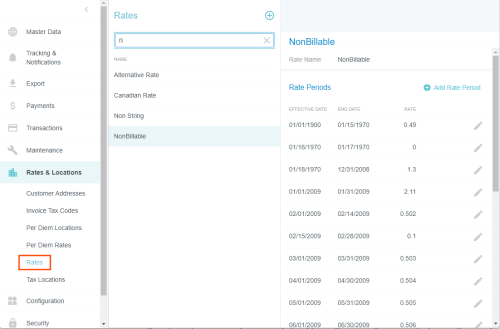## Create a New Rate Period

When the rate is set to change in the future, you may add the new rate period via this screen.

• Note that the end date of the previous rate period will automatically be set to the day before this new rate takes effect—there is no need to edit it.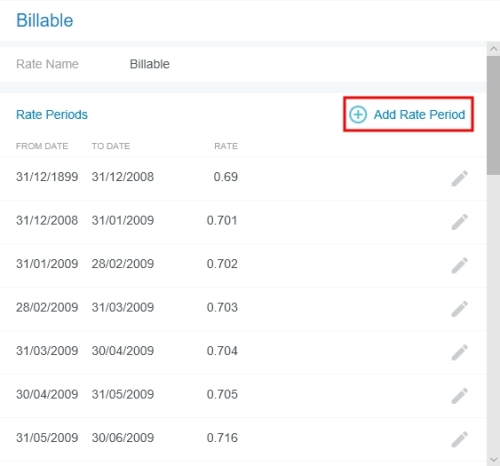2. Enter the From Date (the date that the new rate goes into effect) and the Rate. The To Date is set for you automatically.

Then click SAVE.## Create a New Rate

1. Click the blue plus to add a new rate.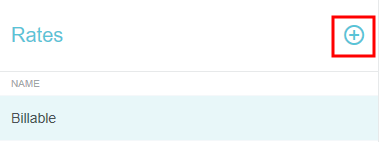2. Give your new rate a name. It cannot match the name of an existing rate, nor may it be edited or deleted once you have saved it.

• If desired, you may set a Currency Code and/or Distance Unit for the rate.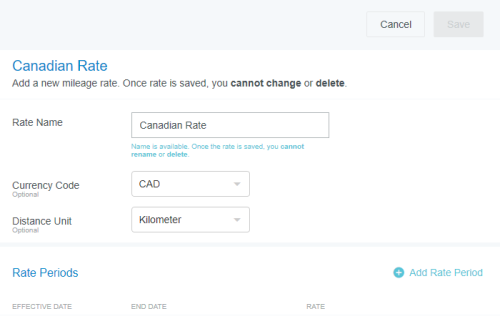3. Now click ADD RATE PERIOD to set the period during which the rate will be valid.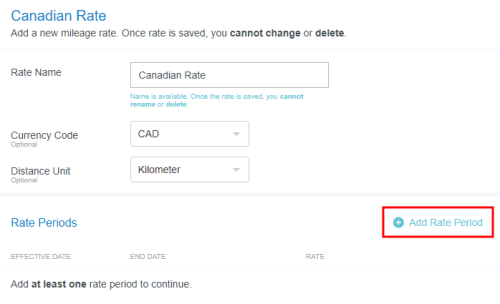4. Select the Effective Date to set the day on which the rate will take effect. Then enter the Rate amount and click SAVE.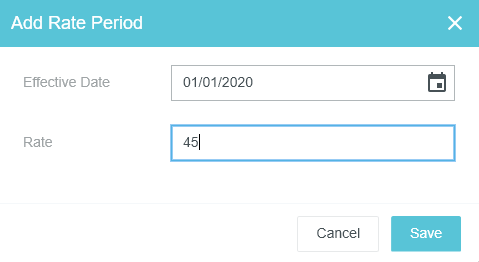5. You may click the pencil icon to make changes, if necessary.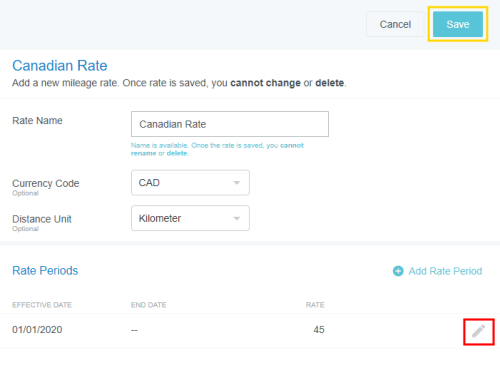6. When you are finished, click SAVE. You will be asked to confirm that you wish to save the rate. Click OK.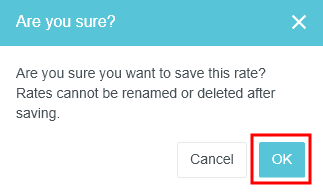## Edit a Rate

1. Click the pencil icon to the right of the rate you wish to edit.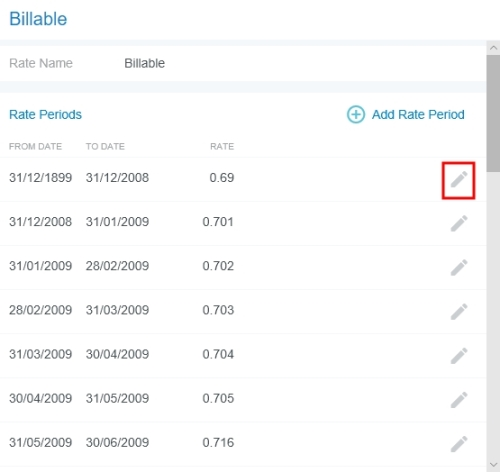2. Note that you may only change the rate, not its name or dates.

Click SAVE.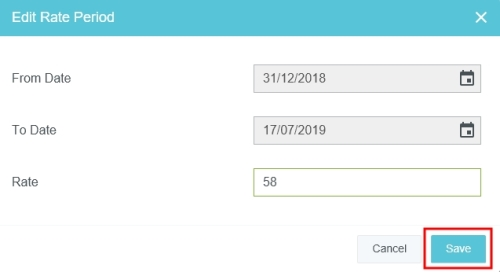<% if (allItems.length > 1) { %>

<% } %>
<% var getColumnClasses = function(numberColumns) { var classNames = 'col-12'; if (numberColumns >= 2) classNames += ' md:col-6'; if (numberColumns >= 3) classNames += ' lg:col-4'; if (numberColumns >= 4) classNames += ' xl:col-3'; return classNames; } %>

##<%= heading %>

<% } %>
<% var getColumnClasses = function(numberColumns) { var classNames = 'col-12'; if (numberColumns >= 2) classNames += ' md:col-6'; if (numberColumns >= 3) classNames += ' lg:col-4'; if (numberColumns >= 4) classNames += ' xl:col-3'; return classNames; } %>

##<%= heading %>

<% } %>
<% var maxSections = 5 %>
<% if (sections.length) { %>
<% sections.forEach(function(section) { %>
• <%= partial('partial-article-list-sections', { id: 'section-' + section.id, parentId: '#' + id, sections: section.sections, activeCategoryId: activeCategoryId, activeSectionId: activeSectionId, activeArticleId: activeArticleId, partial: partial }) %> <% if (section.articles.length) { %> <% } %>
• <% }); %>
<% } %>
<% if (sections.length) { %>
<% sections.slice(0, maxSections).forEach(function(section) { %>
• <%= section.name %> <%= partial('partial-section-list-sections', { parent: section, sections: section.sections, maxSections: maxSections, partial: partial }) %>
• <% }); %> <% if (sections.length > maxSections) { %>
• See more
• <% } %>
<% } %>
<% var getColumnClasses = function(numberColumns) { var classNames = numberColumns === 'auto' ? 'col-auto' : 'col-12'; if (numberColumns >= 2) classNames += ' md:col-6'; if (numberColumns >= 3) classNames += ' lg:col-4'; if (numberColumns >= 4) classNames += ' xl:col-3'; return classNames; } %>
<% blocks.forEach(function(block, index) { %>
• <% if (imageHeight) { %><% } %>
<% if (block.name) { %>

<% if (block.html_url) { %> <%= block.name %> <% } else { %> <%= block.name %> <% } %>

<% } %> <% if (block.description) { %>

<%= block.description %>

<% } %>
• <% }) %>